Skip to content
Related Articles
pandas.concat() function in Python
• Last Updated : 01 Oct, 2020

pandas.concat() function does all the heavy lifting of performing concatenation operations along with an axis od Pandas objects while performing optional set logic (union or intersection) of the indexes (if any) on the other axes.

Syntax: concat(objs, axis, join, ignore_index, keys, levels, names, verify_integrity, sort, copy)

Parameters:

• objs: Series or DataFrame objects
• axis: axis to concatenate along; default = 0
• join: way to handle indexes on other axis; default = ‘outer’
• ignore_index: if True, do not use the index values along the concatenation axis; default = False
• keys: sequence to add an identifier to the result indexes; default = None
• levels: specific levels (unique values) to use for constructing a MultiIndex; default = None
• names: names for the levels in the resulting hierarchical index; default = None
• verify_integrity: check whether the new concatenated axis contains duplicates; default = False
• sort: sort non-concatenation axis if it is not already aligned when join is ‘outer’; default = False
• copy: if False, do not copy data unnecessarily; default = True

Returns: type of objs (Series of DataFrame)

Example 1: Concatenating 2 Series with default parameters.

## Python3

 `# importing the module``import` `pandas as pd`` ` `# creating the Series``series1 ``=` `pd.Series([``1``, ``2``, ``3``])``display(``'series1:'``, series1)``series2 ``=` `pd.Series([``'A'``, ``'B'``, ``'C'``])``display(``'series2:'``, series2)`` ` `# concatenating``display(``'After concatenating:'``)``display(pd.concat([series1, series2]))`

Output: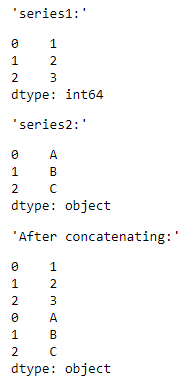Example 2: Concatenating 2 series horizontally with index = 1

## Python3

 `# importing the module``import` `pandas as pd`` ` `# creating the Series``series1 ``=` `pd.Series([``1``, ``2``, ``3``])``display(``'series1:'``, series1)``series2 ``=` `pd.Series([``'A'``, ``'B'``, ``'C'``])``display(``'series2:'``, series2)`` ` `# concatenating``display(``'After concatenating:'``)``display(pd.concat([series1, series2], ``                  ``axis ``=` `1``))`

Output: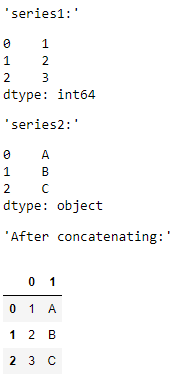Example 3: Concatenating 2 DataFrames and assigning keys.

## Python3

 `# importing the module``import` `pandas as pd`` ` `# creating the DataFrames``df1 ``=` `pd.DataFrame({``'A'``: [``'A0'``, ``'A1'``, ``'A2'``, ``'A3'``], ``                    ``'B'``: [``'B0'``, ``'B1'``, ``'B2'``, ``'B3'``]})``display(``'df1:'``, df1)``df2 ``=` `pd.DataFrame({``'A'``: [``'A4'``, ``'A5'``, ``'A6'``, ``'A7'``], ``                    ``'B'``: [``'B4'``, ``'B5'``, ``'B6'``, ``'B7'``]})``display(``'df2:'``, df2)`` ` `# concatenating``display(``'After concatenating:'``)``display(pd.concat([df1, df2], ``                  ``keys ``=` `[``'key1'``, ``'key2'``]))`

Output: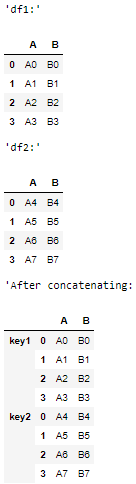Example 4: Concatenating 2 DataFrames horizontally with axis = 1.

## Python3

 `# importing the module``import` `pandas as pd`` ` `# creating the DataFrames``df1 ``=` `pd.DataFrame({``'A'``: [``'A0'``, ``'A1'``, ``'A2'``, ``'A3'``], ``                    ``'B'``: [``'B0'``, ``'B1'``, ``'B2'``, ``'B3'``]})``display(``'df1:'``, df1)``df2 ``=` `pd.DataFrame({``'C'``: [``'C0'``, ``'C1'``, ``'C2'``, ``'C3'``], ``                    ``'D'``: [``'D0'``, ``'D1'``, ``'D2'``, ``'D3'``]})``display(``'df2:'``, df2)`` ` `# concatenating``display(``'After concatenating:'``)``display(pd.concat([df1, df2],``                  ``axis ``=` `1``))`

Output: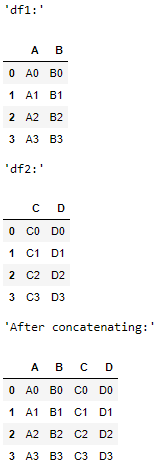Example 5: Concatenating 2 DataFrames with ignore_index = True so that new index values are displayed in the concatenated DataFrame.

## Python3

 `# importing the module``import` `pandas as pd`` ` `# creating the DataFrames``df1 ``=` `pd.DataFrame({``'A'``: [``'A0'``, ``'A1'``, ``'A2'``, ``'A3'``], ``                    ``'B'``: [``'B0'``, ``'B1'``, ``'B2'``, ``'B3'``]})``display(``'df1:'``, df1)``df2 ``=` `pd.DataFrame({``'A'``: [``'A4'``, ``'A5'``, ``'A6'``, ``'A7'``], ``                    ``'B'``: [``'B4'``, ``'B5'``, ``'B6'``, ``'B7'``]})``display(``'df2:'``, df2)`` ` `# concatenating``display(``'After concatenating:'``)``display(pd.concat([df1, df2], ``                  ``ignore_index ``=` `True``))`

Output: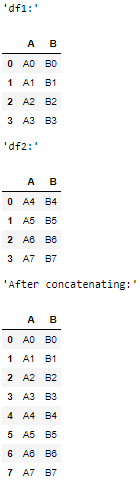Example 6: Concatenating a DataFrame with a Series.

## Python3

 `# importing the module``import` `pandas as pd`` ` `# creating the DataFrame``df ``=` `pd.DataFrame({``'A'``: [``'A0'``, ``'A1'``, ``'A2'``, ``'A3'``], ``                    ``'B'``: [``'B0'``, ``'B1'``, ``'B2'``, ``'B3'``]})``display(``'df:'``, df1)``# creating the Series``series ``=` `pd.Series([``1``, ``2``, ``3``, ``4``])``display(``'series:'``, series)`` ` `# concatenating``display(``'After concatenating:'``)``display(pd.concat([df, series],``                  ``axis ``=` `1``))`

Output: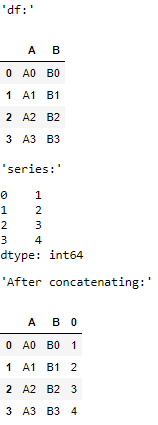Attention geek! Strengthen your foundations with the Python Programming Foundation Course and learn the basics.

To begin with, your interview preparations Enhance your Data Structures concepts with the Python DS Course. And to begin with your Machine Learning Journey, join the Machine Learning – Basic Level Course

My Personal Notes arrow_drop_up